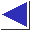# comp.lang.c FAQ list ·Question 7.8

Q: I see code like

```	char *p = malloc(strlen(s) + 1);
strcpy(p, s);
```
Shouldn't that be malloc((strlen(s) + 1) * sizeof(char))?

A: It's never necessary to multiply by sizeof(char), since sizeof(char) is, by definition, exactly 1. (On the other hand, multiplying by sizeof(char) doesn't hurt, and in some circumstances may help by introducing a size_t into the expression; see question 7.15.) See also questions 8.9 and 8.10.

References: ISO Sec. 6.3.3.4
H&S Sec. 7.5.2 p. 195